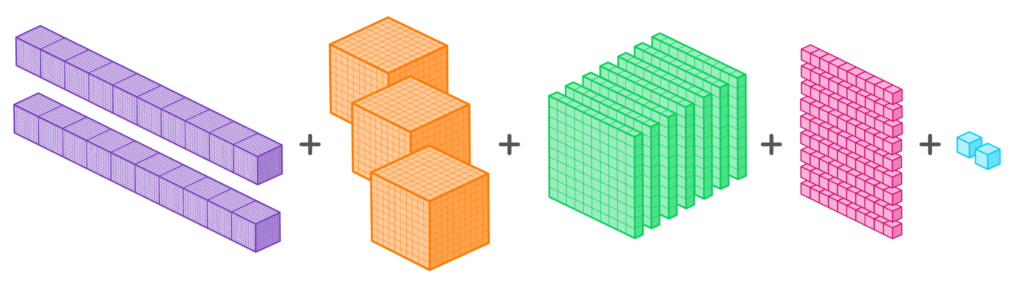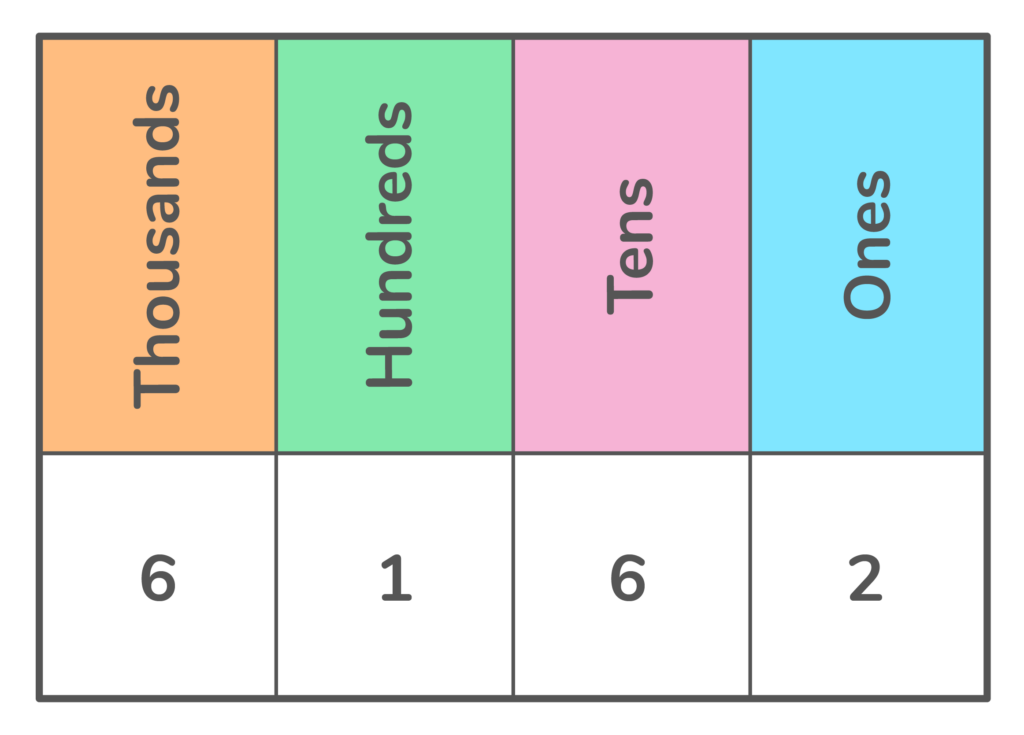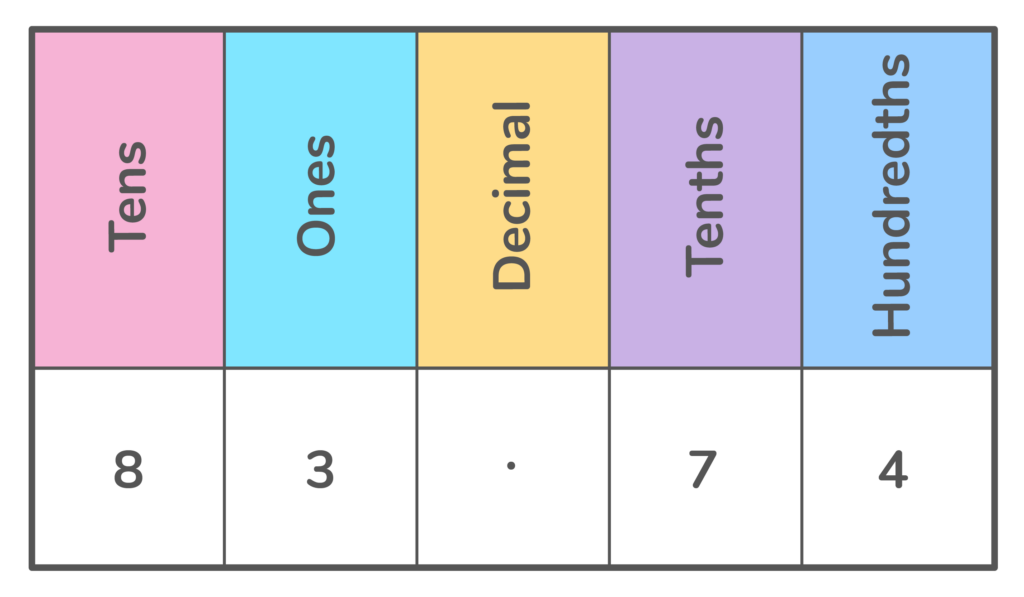# What is Expanded Form?

Learn what standard form is and have a go at some questions!Author
Katie WickliffPublished
October 25, 2023# What is Expanded Form?

Learn what standard form is and have a go at some questions!Author
Katie WickliffPublished
Oct 25, 2023# What is Expanded Form?

Learn what standard form is and have a go at some questions!Author
Katie WickliffPublished
Oct 25, 2023

Key takeaways

• Expanded form is a way to write numbers by adding the value of its digits
• Using expanded form helps us to determine place value and add up large numbers without using a calculator!

As students learn about the place value of numbers, they also learn about expanded form. If you don’t know what expanded form means, don’t worry! This article provides a comprehensive overview of expanded form and explains how to write numbers in expanded form. We also include plenty of examples and practice problems to build your student’s confidence. Let’s get started!

## What is Expanded Form in Math?Expressing a number in expanded form stretches out the number to show the value of each digit. While we are used to seeing numbers in their standard form, expanded form helps determine place value and is also a way to add large numbers without a calculator. Understanding expanded form helps students develop stronger number sense.

## Examples of Expanded FormStandard Form: 834

8 hundreds

3 tens

4 ones

Expanded Form: 800+30+4

In this expanded form example, we see that the digit in the hundreds place (8) is represented by writing 800, the digit in the tens place (3) is represented by writing 30, and the digit in the ones place (4) is represented by writing 4.

Let’s try another expanded form example with a larger number:

Standard Form: 23,791

2 ten thousands

3 thousands

7 hundreds

9 tens

1 ones

Expanded Form: 20,000+3,000+700+90+1## How to Write Numbers in Expanded FormUnderstanding place value is essential to writing numbers in their expanded form. Using a place value chart or another table can help students accurately express the expanded form of both whole numbers and decimal numbers, like the example below:

### Writing Whole Numbers in Expanded FormStart from the left with the first digit (6). We know that the digit is in the thousands place, so write “6” in the blank space under thousands. Then we do the same for the digit in the hundreds place, in the tens, and in the ones.

Inserting digits into the chart helps students write the whole number in expanded form:

6,000+100+60+1### Writing Decimals in Expanded FormDecimal numbers consist of a whole number and a fractional part, separated by a dot called a decimal point.  In a decimal number, the digits to the left of the decimal point are whole numbers, and the digits to the right of the decimal point are fractional parts of the number. Writing expanded form with decimals is similar to writing whole numbers in expanded form. Students can use a place value chart or table to write the expanded form of decimals, like the example below:

Standard Form: 83.74Inserting digits into the chart helps students write the decimal number in expanded form:

Expanded Form:

80+3+7/10 + 4⁄100

Or

80+3+0.7+0.04

## Practice problems

700,000+20,000+3,000+100+10+9

40+5+0.7+0.02

645Expanded form is a method of writing a number to show the value of each digit.

You can write a number in expanded form by writing the place value of each digit and then adding them together to reach the sum in the standard form.Lesson credits

Katie Wickliff

Katie holds a master’s degree in Education from the University of Colorado and a bachelor’s degree in both Journalism and English from The University of Iowa. She has over 15 years of education experience as a K-12 classroom teacher and Orton-Gillingham certified tutor. Most importantly, Katie is the mother of two elementary students, ages 8 and 11. She is passionate about math education and firmly believes that the right tools and support will help every student reach their full potential.

Katie Wickliff

Katie holds a master’s degree in Education from the University of Colorado and a bachelor’s degree in both Journalism and English from The University of Iowa. She has over 15 years of education experience as a K-12 classroom teacher and Orton-Gillingham certified tutor. Most importantly, Katie is the mother of two elementary students, ages 8 and 11. She is passionate about math education and firmly believes that the right tools and support will help every student reach their full potential.# Are you a parent, teacher or student?

Are you a parent or teacher?

## Hi there!

Book a chat with our team